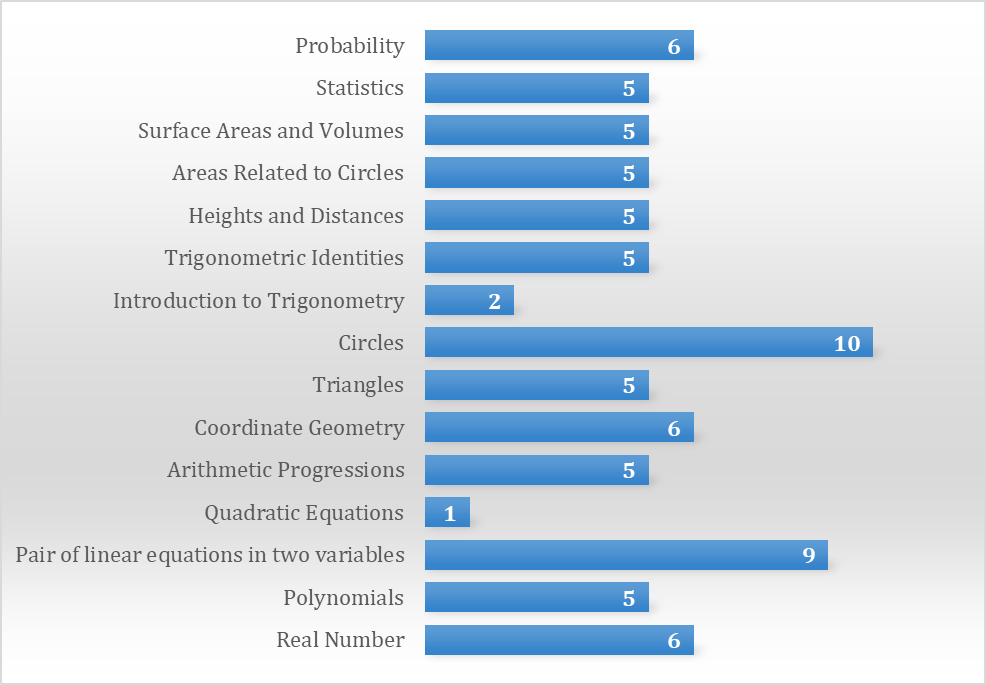Request a call back

# CBSE Class 10 Board Exam 2023 Post Paper Analysis - Maths (21st March)The Central Board of Secondary Education (CBSE) successfully conducted the Mathematics Theory Paper for Class 10 today, 21st March 2023.

This time, CBSE has returned with its annual exam pattern based on the syllabus. So, let’s go through the paper pattern in detail.

The paper was 80 marks (comprising a total of 38 questions), which students were expected to attempt in three hours. The question paper was divided into five sections A, B, C, D and E.

Section A had 20 objective types of questions, each carrying 1 mark with a combination of MCQs and Assertion – Reasoning-based questions.

Section B had 5 VSAQs, each carrying 2 marks with a combination of reasoning and application-oriented questions.

Section C had 6 SAQs, each carrying 3 marks with a combination of numerical-based and HOTs type of questions.

Section D consisted of 4 LAQs carrying 5 marks each.

Section E consisted of 3 case-based integrated units of assessment of 4 marks, each with sub-parts.

All the questions were compulsory to attempt. However, an internal choice was provided in some questions where students were expected to try only one of these questions.

Here we decode the CBSE Class 10 Math Paper for 2023 with the final review and analysis for you.

## Chapter-wise Distribution of MarksCircles had the highest marks, and only three questions were asked from this chapter. In comparison, the chapter Quadratic Equations had given the least weightage. According to students' reactions and experts' feedback, the basic paper was easy but a little lengthy. However, it was manageable so that students could complete it within the allotted time. At the same time, the standard paper was well-balanced, and the questions were based entirely on NCERT.

As per students and experts, the paper's difficulty level was moderate and covered all important topics. Also, there was a mixture of easy and tricky questions. The paper's structure was precisely like the Maths Sample Paper, and the difficulty level was similar. However, if the students have thoroughly gone through the NCERT textbooks, they can quickly solve the questions.

## Here is the section-wise difficulty level for CBSE 10 Math Paper:

 Parameter Difficulty Level Section A Easy Section B Easy Section C Tricky Section D Tricky Section E Easy

So, sections A, B and E were easy to moderate, whereas sections C and D were a bit tricky but could be solved if a student was well-versed with the NCERT. The case study questions required a thorough understanding of the concepts.

We, too, agree that the Math paper was moderate, with few tricky and direct questions. However, writing the step-by-step solutions would have taken a long time.

## Watch CBSE Class 10 Maths Post Paper Analysis detailed explanation-

For any exam-related help and queries, you can visit TopperLearning. For more paper reviews and exam updates, stay tuned.

Previous Article
Next Article